### Home > CALC > Chapter 3 > Lesson 3.4.3 > Problem3-176

3-176.
1. Find the following limits quickly. (Hint: Review your solution for problem 3-92 first!) Homework Help ✎

1.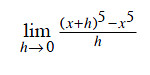2.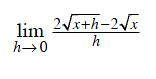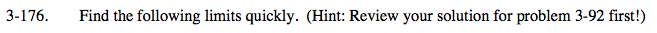These are both Hana's definition of the derivative. Instead of algrabraically finding these limits (as you did in 3-175), you could deconstruct her definition and apply the power rule.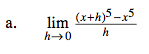Hana's definition of the derivative:

$f'(x) =\lim_{h\rightarrow 0}\frac{f(x+h)-f(x)}{h}$

What if f(x)? f(x) = x5
What if f '(x)? f '(x) = 5x4

$=\lim_{h\rightarrow 0}\frac{(x+h)^5-x^5}{h}$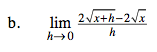Hana's definition of the derivative:

$f'(x) =\lim_{h\rightarrow 0}\frac{f(x+h)-f(x)}{h}$

What if f(x)? f(x) = ___________
What if f '(x)? f '(x) = ___________

$=\lim_{h\rightarrow 0}\frac{2\sqrt{x+h}-2\sqrt{x}}{h}$